### Introduction:

This is the forth SAP2000 tutorial, at the end of this tutorial you'll be able to make a full structural analysis of any beam contains internal hinges with more complicated beams that we took in SAP2000 tutorial No. 3, and to display shear and moment diagrams as well.

### Defining internal hinges

Internal hinges can easily be defined using SAP after understanding it is effect at structural system. When a system contains a hinge user will expect zero moment at that point.

Example 1: Draw the shear and moment diagrams for the compound beam which is pin connected at the midpoint between the supports.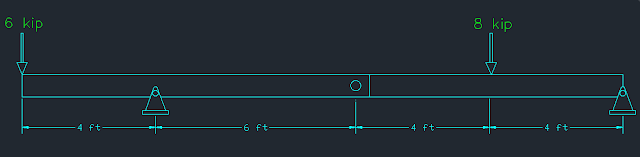[Fig.1]

1- Draw the system using SAP. Make sure you draw two separate parts beside B (B as defined on problem not the bubble).

2- Select the part to the left to the internal hinge as shown in figure 2.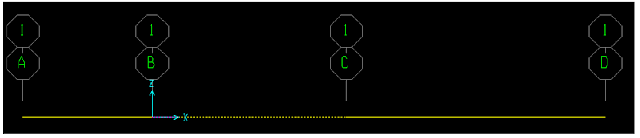[Fig.2]

3- Assign ➥ Frame ➥ Releases /partial fixity (see figure 3).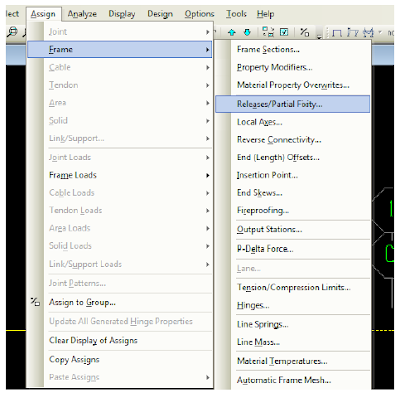[Fig.3]

4- Since the function of internal hinge is to make a point free to rotate then it is assumed that moment value there will be zero (inflection point). To make moment value zero at the end of the selected line ( End or start: according to your way of drawing; if you started your drawing from

right to left then it is the end. If you do the opposite thing the left part of the line is the start. In our case it is the end.

5- Check M33 – End. (See figure 4) then press ok.[Fig.4]

6- after clicking on OK you must see the same result shown in figure 5.

SAP trims the end of the beam just to indicate you that a change in releases options took place. No real changes indeed.[Fig.5]

7- Repeat the same procedure to part to the right of point C: select beam then the same steps explained previously in point 3. But this time check Start instead of End (see figure 6).[Fig.6]

8- Run the model but before doing this remember that this type of problems assume no self-weight (change self-weight multiplayer to zero for the dead load pattern).

9- Display the shear and moment diagram, and see how moment value is zero where you changed moment releases (see figure 7).[Fig.7]

### Definition of points displacements:

SAP2000 software can easily define settlements at certain points. Determinant systems results do not change after defining settlements of points. Indeterminate systems results change.

Example: For the simply supported beam (5 meter length) shown in figure 8 define two displacements values (3 cm at point A, 5 cm at point B).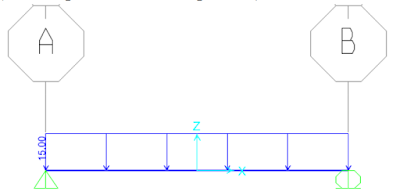[Fig.8]

1-Select point at A.
2- Assign ➥ Joint loads ➥ displacements (see figure 9).[Fig.9]

3- Since displacement is in z direction, change value besides “Translation Global Z” to -3 (note that units to the right is chosen in cm) [see figure 10].[Fig.10]

4- Repeat the same procedure for point B.

User can defined many load patterns in SAP2000 software. These load patterns can be gathered through what is known “Load combination”. If user are working using two load patterns “Dead and live, a two load combination can be defined:

1- Define ➥ Load combinations (see figure 11).[Fig.11]

2- Click on “add new combination”. (See figure 12).[Fig.12]

3- Fill the blanks as shown in figure 13.[Fig.13]

4- to add load combination 2 do the same procedure mentioned previously. Make sure you have the same input as shown in figure 14.[Fig.14]

5- To define envelope load combination make sure you have the same input as shown in figure 15.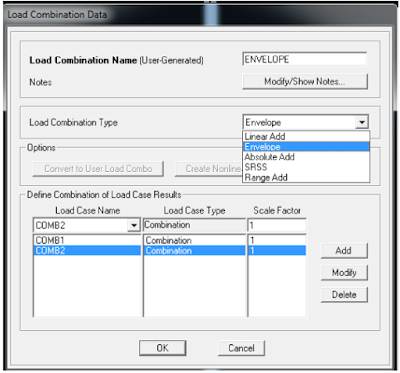[Fig.15]

6- The final result will be as shown in figure 16.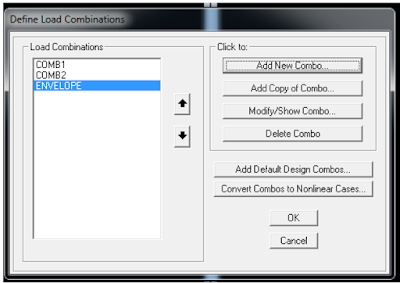[Fig.16]

### Definition of beams using beams template.

Example (from assignments):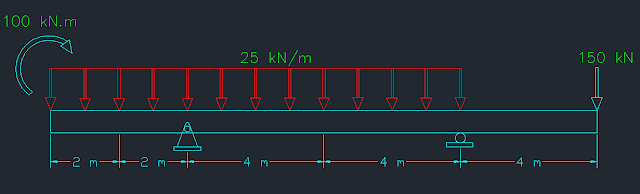[SKETCH]

Define 3 load combinations (mentioned previously).

1- Check your units (KN, m) before opening a new file on SAP.
2- Choose “Beam” template from options. (See figure 17).[Fig.17]

2- in number of spans type: 3, and since spacing is not uniform we will not care about spacing option (keep as default).
3- Check option: use custom grid spacing and locate origin (see figure 18).[Fig.18]

4- now click on: Edit grid option and modify values according to our problem (see figure 19).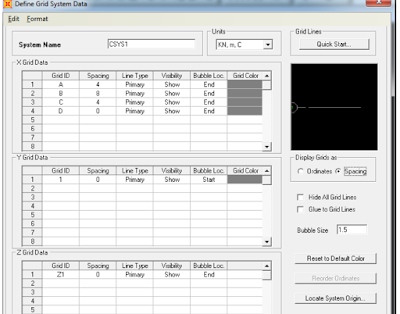[Fig.19]

5- after click ok we will get window shown in figure 18. Note we can determine the beam section if self-weight to be included through solution (or for deflections calculations). In our problem self-weight is to be ignored so nothing will be changer here.

6- If you follow the instructions an output as shown in figure 20.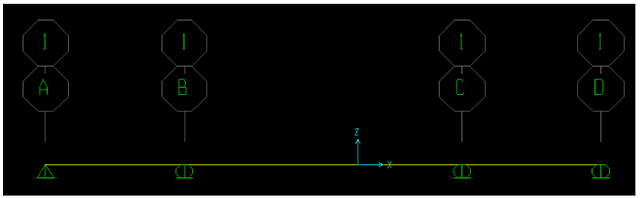[Fig.20]

Note that this system needs some modifications to be as required in the problem.

7- To make A & D ends free do the following steps:

Select both points by clicking on them ➥ assign ➥ joints ➥ from fast restraints choose the last option (free point) [see figure 21].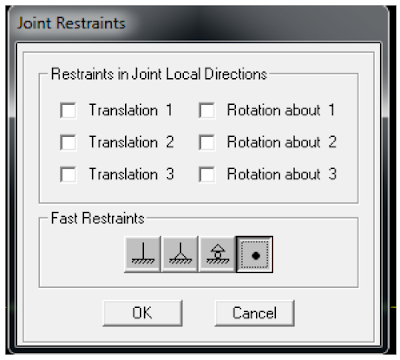[Fig.21]

8- change B & C supports to be pin, roller supports respectively.
9- Define live load pattern (mentioned previously on handout #2).[Fig.22]

11- To display loads on beams to ensure that you are working correctly you can do one of these two ways:

a) Click on display ➥ show load assigns (see figure 23) ➥ choose type of load to display (joint, or frame) and check the loads.[Fig.23]

b) Select any beam by clicking on it, then right click by mouse (see figure 23).[Fig.24]

12- Analyze the system after saving the file.

13- Display moment and shear diagram many times (once for dead load only, once for live load …etc.).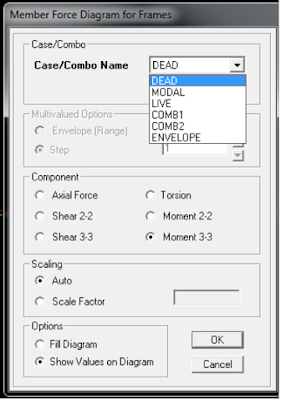[Fig.25]

#### 1 comment:

1.